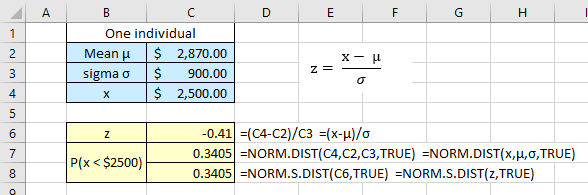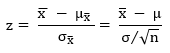# Normal Distribution Problems- Two Common Mistakes

I see many students in my intro statistics courses missing problems related to the normal distribution.

Common Mistake #1: One especially common mistake is not using the correct “standard deviation” to find probabilities and percentiles.

Consider the following problem statement:

A bank auditor claims that credit card balances are normally distributed, with a mean of \$2870 and a standard deviation of \$900.

1. What is the probability a randomly selected credit card holder has a card balance less than \$2500?
2. You randomly select 25 credit card holders. What is the probability that their mean card balance is less than \$2500?
3. Interpret the two probabilities in terms of the auditor’s claim.

I usually see students get one of the questions correct, but not all. And they either seem to get #1 or #2 correct in about equal proportions. When I inspect their solutions, I find that they get confused over the “standard deviation” to use in the equation for z.

Most students seem to get #1 correct. They use the formula for z:correctly interpreting the problem’s “standard deviation of \$900” as the population sigma.

Here is the Excel solution for part 1. Note, I give two formulas for finding the probability. In both, “True” gives the cumulative probability from left infinity; the left tail, in other words.Here is the StatCrunch solution using the Stat > Calculators > Normal command sequence. In the dialog box, make sure the Standard option is active, enter the mean, sigma, x, and select the < to get the left tail, and then click Compute. I like to use StatCrunch for these types of problem since it gives a sketch as well as the probability.In both solutions to part 1, we find that the probability of an individual card holder having less than a \$2500 balance is 34%, which is not unusual.

Let’s look at part 2 again:

1. You randomly select 25 credit card holders. What is the probability that their mean card balance is less than \$2500?

The mistake I see many students make is to use the population sigma in their calculations. That means they probably did not recognize that the question is about a mean for 25 randomly selected card holders. In other words, a sample.

To find z for a sample, you must use the standard deviation of the sampling distribution of sample means, the standard error, σ.This is the formula for finding z for a sample mean, x̅:Recall that the mean of a sampling distribution of sample means, µx̅ , is the population mean, µ.

Here is the Excel solution:Here is the StatCrunch solution, again using the Normal calculator.

I just learned a neat “trick” about the calculator: you can use Excel-like formulas in the data entry windows. Here, to get the standard error, I entered 900/SQRT(25) in the Std. Dev. Window before I clicked Compute. Of course, you can use a regular calculator to find the standard error and enter that value.In the StatCrunch graph, we can see that the \$2500 sample mean balance is very far to the left of the population mean of \$2870. Thus, the approximately 2% chance of getting less than \$2500 for a sample of 25 is reasonable.

But getting a sample mean of \$2500 for this population would be unusual if our standard of labeling an event unusual is a 5% chance.

Common Mistake #2: Another common mistake on a similar problem but with a key difference in the wording.

Use the normal distribution of fish lengths for which the mean is 11 inches and the standard deviation is 4 inches. Assume the variable x is normally distributed.

1. What percent of the fish are longer than 14 inches?
2. If 200 fish are randomly selected, about how many would you expect to be shorter than 9 inches?

Part 1 is straightforward. We are asked about individual fish, not a sample.

This time I will use StatCrunch first so we can see the sketch.We can see that 14 inches is to the right side of the mean of 11 inches. The area under the normal curve to the right of 14 is 0.2266 which means that about 22.7% of the fish will be longer than 14 inches.

This is the Excel solution:Because we need the right tail, we must subtract the value returned by the NORM.S.DIST function from one. Recall the TRUE parameter gives us the cumulative area under the curve from left infinity to our z value. Unfortunately, “False” does not give the right tail!

Students who do not draw the sketch often forget this important step and give an incorrect answer of 77.3%.

Part 2: If 200 fish are randomly selected, about how many would you expect to be shorter than 9 inches?

I think what throws some students on part 2 is the statement “If 200 fish are randomly selected” which sounds an awful lot like it is a sample. And it is a sample of n = 200.

But the key is that they do not ask for the mean or any other sample statistic.

They want to know how many of the 200 fish will be shorter than 9 inches. That means we do not need to use the standard error, σ. We should again use sigma as the standard deviation in the StatCrunch normal calculator.

We get a probability of 30.9%, which means about 62 [200*30.9%] of the fish will be shorter than 9 inches.Here is the Excel solution:Because we are again interested in the left tail (see the StatCrunch sketch), we go back to our original formulas by deleting the “1-” in front of the NORM.S.DIST function. And, as with StatCrunch, we see that about 62 fish of the 200 will be shorter than 9 inches in length.

### 2 thoughts on “Normal Distribution Problems- Two Common Mistakes”

1.Great.But to follow your explanation requires excel knowledge and mental effort.{this is not meant to be a negative comment.

1.Yes, this post was originally aimed at students in my intro stats class. They must pick a technology to use with the course. Most use either Excel or StatCrunch.

This site uses Akismet to reduce spam. Learn how your comment data is processed.